Win up to 100% scholarship on Aakash BYJU'S JEE/NEET courses with ABNAT Win up to 100% scholarship on Aakash BYJU'S JEE/NEET courses with ABNAT

# Constrained Motion

The restrictions on the motion, position or both motion and position of an object are called constraints. The elements of the machine will form a pair if they are in contact with each other. The pair is called a kinematic pair if the motion between the pair is completely or partially constrained.

## What Is a Constrained Motion?

The body has six degrees of freedom when it is not constrained. The body will not move in any direction if all the degrees of freedom are locked. The motion that occurs when the body is allowed to move in one direction but constrained in all the other directions is called constrained motion. In a constrained motion, the object moves in a restricted way. For example, the circular motion of the fan is a constrained motion.

The force exerted by the constraining object on a particular object to make it follow the constraints of the movement is known as the constrained force.

## Types of Constrained Motion

There are three types of constrained motion:

1. Completely constrained motion
2. Partially or successfully constrained motion
3. Incompletely constrained motion

### Completely Constrained Motion

Completely constrained motion is when the motion of the pair is limited to one direction, irrespective of the direction of the applied force. For example, a rectangular shaft moving in one direction in a rectangular hole is a completely constrained motion. The shaft cannot rotate or move in any other direction.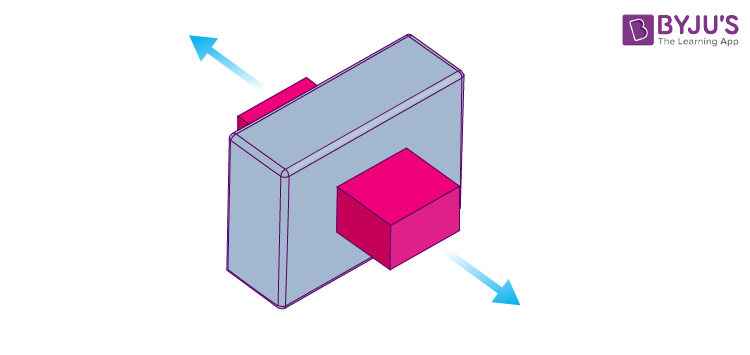### Partially or Successfully Constrained Motion

In partially constrained motion, when there is no external force applied, the motion will be in more than one direction, but when an external force is applied, the motion is restricted to a single direction. Partially constrained motion is also called successfully constrained motion. The footstep bearing moves in only one direction when an external force is applied, so it is an example of partially constrained motion.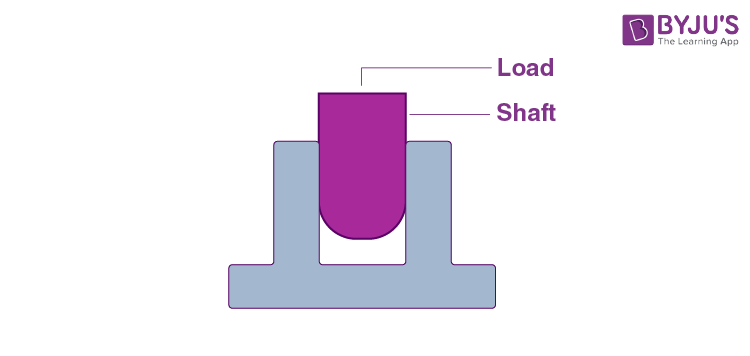### Incompletely Constrained Motion

In an incompletely constrained motion, the motion between the pair can take place in more than one direction. A circular shaft moving in a circular hole is an example of incompletely constrained motion.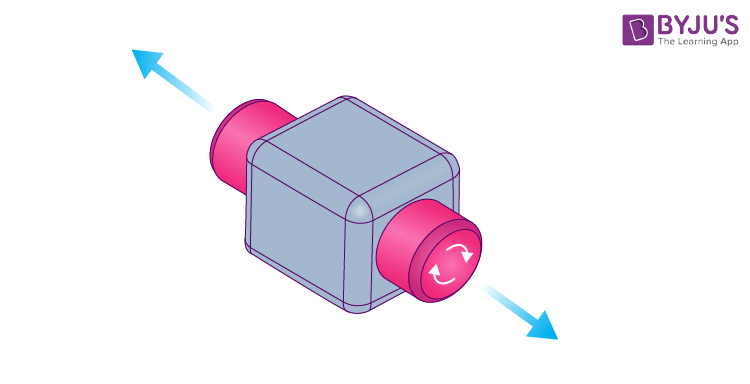## Solved Examples

1. In the system shown in the figure below, if block A is pulled towards the right at a constant speed of 1 m/s, find the speed of block B w.r.t. the ground.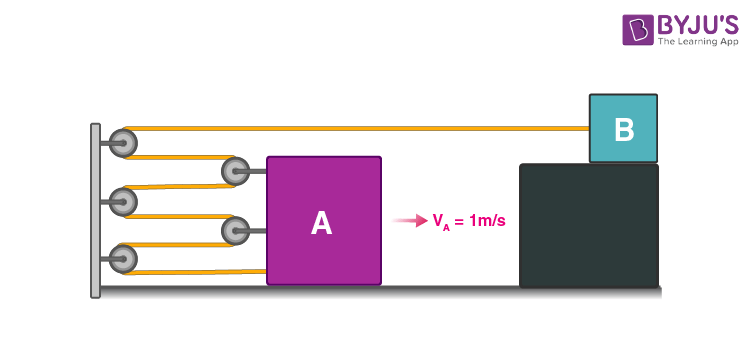1. 1 m/s
2. 3 m/s
3. 4 m/s
4. 5 m/s

Since there is no external force applied, the work done will be zero.

$$\begin{array}{l}\sum \vec{T}.\vec{V}=0\end{array}$$

⇒ 5 T. (-1) + T.V = 0

⇒T.V = 5T

V = 5 m/s

2. In the arrangement shown below, neglect the mass of the ropes and pulley. What must be the value of ‘m’ to keep the system in equilibrium? (There is no friction).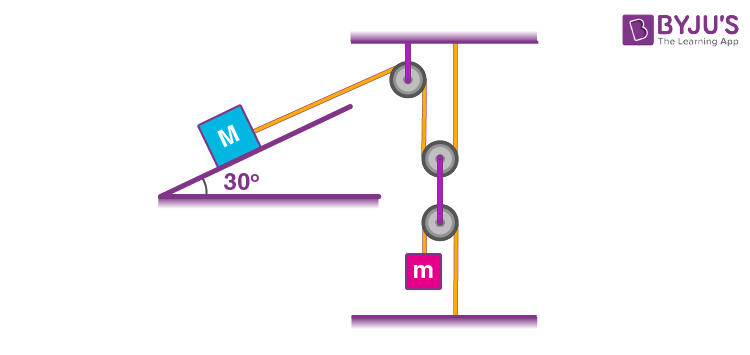Solution: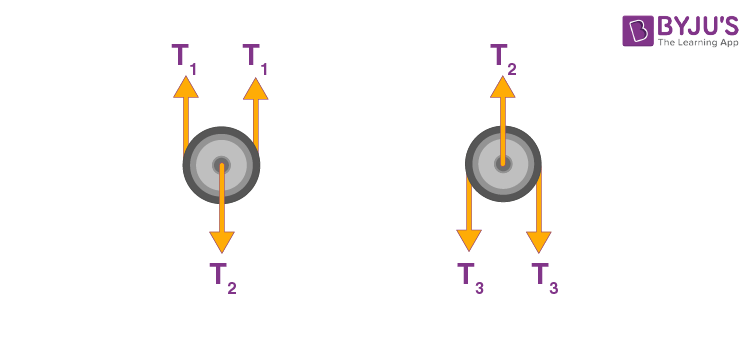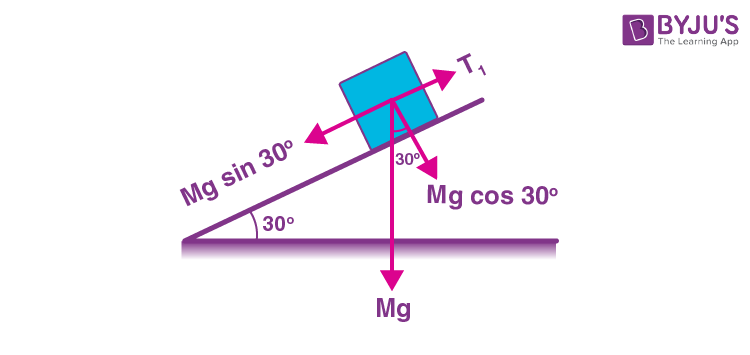T1 = Mgsin 300 = Mg/2 ——-(1)

2T1 = T2 and T2 = 2T3 ——-(2)

For equilibrium of mass m, mg = T3 ———(3)

From equations (1), (2) and (3), we get

mg = Mg/2

⇒ m = M/2

3) Consider a system of three equal masses and 4 pulleys arranged, as shown in the figure below. The mass of each block is taken as m. Find the acceleration of B and C and the tension of the string.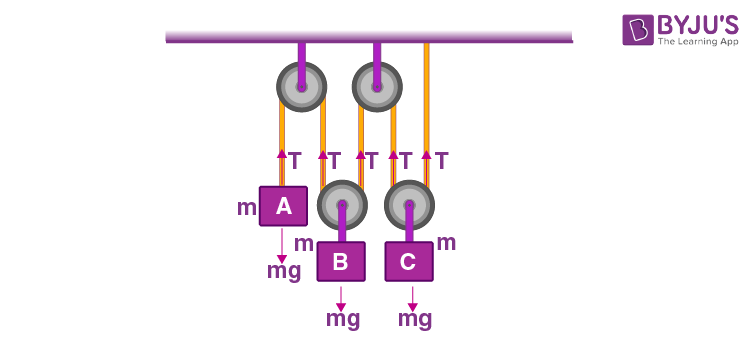Solution:

By constraint equation,

aA + 2aB + 2aC= 0

aB = aC

Therefore , aA + 4aB = 0

Assuming aA = a and aB = a1

The downward motion equation of block A

mg – T = ma ——-(1)

The downward motion equation of block B

2T – mg = ma1——-(2)

Solving (1) and (2)

mg = 2ma + ma1

= 2m(4a1) + ma1

a1 = (g/9) m/s2

⇒ aB = aC = g/9 m/s2

From equation (2), we have

2T = mg + ma1

= mg + mg/9

2T = 10mg/9

T = (5/9)mg

4) Two masses, m1 =1 kg and m2 = 2 kg, are connected by a light inextensible string and suspended by means of a weightless pulley, as shown in the figure below. Assuming that both the masses start from rest, what is the distance travelled by the centre of mass in 2 s? (take, g = 10 m/s2).a. 20/9) m

b. (40/9) m

c. (⅔) m

d. (⅓) m

Solution:

Here, m1 = 1 kg, m2 = 2 kg

The acceleration of the system is

$$\begin{array}{l}a = \frac{(m_{2}-m_{1})g}{m_{1}+m_{2}} = \frac{(2-1)g}{1+2}= g/3= 10/3\end{array}$$
$$\begin{array}{l}a_{com}=\frac{\frac{10}{3}\times 1-\frac{10}{3}\times 2}{3}\end{array}$$

= (-10/9) m/s2

s = ut + (½)at2

s = 0 + (½) (10/9) x 22

= 20/9 m

Related Video